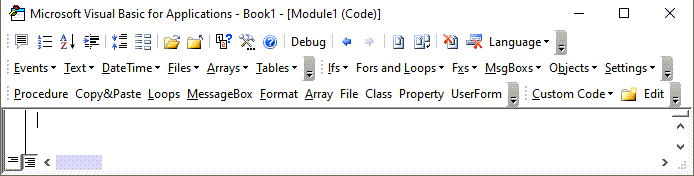# VBA CLng Function – Convert Expression to Long

This tutorial will demonstrate how to use the CLng VBA function to convert an expression to the long integer data type.

## CLng Function

### VBA CLng Convert Expression to Long

The VBA CLng function can be used to convert expressions to long data type inside the VBA code.

``````Sub CLngExample_1()
MsgBox CLng(12.34)  'Result is: 12
MsgBox CLng(12.345) 'Result is: 12
MsgBox CLng(-124)   'Result is: -124
MsgBox CLng(-12.34) 'Result is: -12
End Sub
``````

### VBA CLng Rounding

The VBA CLng function will round the decimal part of a number type or a number like expression. However, it does not round correctly in all cases. When the decimal part is 0.5 then VBA CLng function returns the closest even integer.

``````Sub CLngExample_2()
MsgBox CLng(0.34)     'Result is:    0

MsgBox CLng(0.99)     'Result is:    1

MsgBox CLng(-124.95)  'Result is:    -125

MsgBox CLng(1.5)      'Result is:    2

MsgBox CLng(2.5)      'Result is:    2
End Sub
``````

We can add a decimal number relatively small to our expected decimal value to change the behavior of VBA CLng function to the expected.

``````Sub CLngExample_3()
MsgBox CLng(2.5)
'Result is:    2
MsgBox CLng(2.5 + 0.001)
'Result is:    3

MsgBox CLng(14.5)
'Result is:   14
MsgBox CLng(14.5 + 0.001)
'Result is:   15
End Sub
``````

### VBA CLng Converting Strings to Longs

The VBA CLng function can be used to convert strings to longs if the characters in the string have a meaning as numbers.

``````Sub CLngExample_4()
Dim StrEx As String
StrEx = "112"
MsgBox CLng(StrEx)
'Result is: 112

StrEx = "112.3"
MsgBox CLng(StrEx)
'Result is: 112        112.3 is rounded

StrEx = "11,2"
MsgBox CLng(StrEx)
'Result is: 112        , is ignored

StrEx = "\$112"
MsgBox CLng(StrEx)
'Result is: 112        \$ is ignored
End Sub
``````

### VBA CLng Run-Time Error 13 Type Mismatch

Using VBA CLng function with strings that contain non-numerical characters or characters that don’t have meaning in numerical context will result in a Run-Time error ’13’: Type mismatch.

``````Sub CLngExample_5()
'The code below will result in an ERROR message
'CLng can’t handle non numerical characters
Dim StrEx As String
StrEx = "Ab13"
MsgBox CLng(StrEx)
End Sub
``````

### VBA CLng Run-Time Error 6 Overflow

Using VBA CLng function with strings that result in a value smaller or bigger than the expected integer will result in a Run-Time error ’6’: Overflow. Integer data type in excel has an expected value of -2,147,483,648 to 2,147,483,647.

``````Sub CLngExample_6()
'The code below will result in an ERROR message
'CLng cant handle non numerical characters
Dim StrEx As String
StrEx = "2147483648"
MsgBox CLng(StrEx)
End Sub
``````

Stop searching for VBA code online. Learn more about AutoMacro - A VBA Code Builder that allows beginners to code procedures from scratch with minimal coding knowledge and with many time-saving features for all users!### VBA CLng Regional Settings

VBA CLng function has different behavior converting strings with comma or dot.  It uses the Regional Settings of the operating system for decimal separator and digit separator.

``````Sub CLngExample_7()
Dim StrEx As String
StrEx = "1,9"
MsgBox CLng(StrEx)
‘If Regional settings have , as a grouping separator then
'Result is: 19
‘If Regional settings have , as a decimal separator then
'Result is: 2 (2 because 1.9 gets rounded)

StrEx = "1.9"
MsgBox CLng(StrEx)
‘If Regional settings have . as a grouping separator then
'Result is: 19
‘If Regional settings have . as a decimal separator then
'Result is: 2 (2 because 1.9 gets rounded)
End Sub
``````

### VBA CLng Converting Booleans to Longs

VBA CLng function can convert boolean variables to longs. If the evaluated expression is true the resulting long is -1 and if the evaluated expression is false the resulting long is 0.

``````Sub CLngExample_8()
Dim BoolEx As Boolean
BoolEx = True
MsgBox CLng(BoolEx)
'Result is: -1
MsgBox CLng(2 = 2)
'Result is: -1

BoolEx = False
MsgBox CLng(BoolEx)
'Result is: 0
MsgBox CLng(1 = 2)
'Result is: 0
End Sub
``````

### VBA CLng Converting Dates to Longs

VBA CLng function can convert a date variable to a long. The returned value is the internal number used by excel for date storage rounded. If that number is outside of the expected long limits for VBA then we get a Run-Time error ’6’: Overflow.

``````Sub CLngExample_9()
Dim DateEx As Date
DateEx = #2/3/1940#
MsgBox CLng(DateEx)
'Result is: 14644
DateEx = #8/7/1964#
MsgBox CLng(DateEx)
'Result is: 23596
DateEx = #3/7/1934 11:32:04 AM#
MsgBox CLng(DateEx)
'Result is: 12485
End Sub
``````